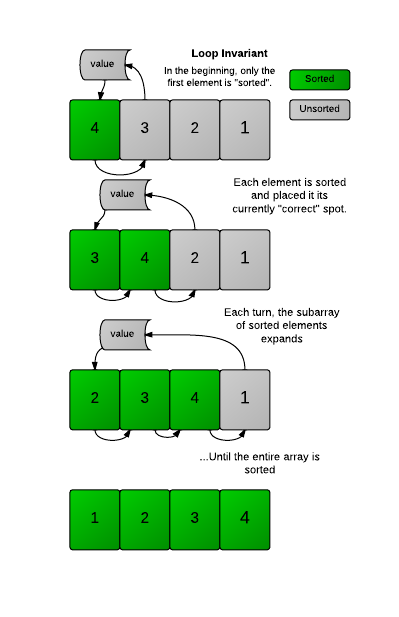# Correctness and the Loop Invariant

In the previous challenge you wrote code to perform an Insertion Sort on an unsorted array. But how would you prove that the code is correct? I.e. how do you show that for any input, your code will provide the right output?

Loop Invariant
In computer science, you could prove it formally with a loop invariant, where you state that a desired property is maintained in your loop. Such a proof is broken down into 3 parts:

• Initialization - It is true (in a limited sense) before the loop runs.
• Maintenance - If it's true before an iteration of a loop, it remains true before the next iteration.
• Termination. It will terminate in a useful way, once it is finished.

Insertion Sort's Invariant
Say you have some InsertionSort code, where the outer loop goes through the whole array A:

``````for(int i = 1; i < A.length; i++){
//insertion sort code
``````

You could then state the following loop invariant:

At the start of every iteration of the outer loop (indexed with i) the subarray until ar[i] consists of the original elements that were there, but in sorted order.

To prove Insertion Sort is correct, you will then demonstrate it for the three stages:

• Initialization - The subarray starts with the first element of the array, and it is (obviously) sorted to begin with.

• Maintenance - Each iteration of the loop expands the subarray, but keeps the sorted property. An element V only gets inserted into the array when it is >= to the element to the left of it. Since the elements to the left have already been sorted, it means V >= all the elements to the left, so the array remains sorted. (In InsertionSort2, we saw this by printing array each time an element was 'inserted' into it.)

• Termination - The code will terminate after i has reached the last element in the array, which means the sorted subarray has expanded to encompass the entire array. So the array is now fully sorted.You can often use a similar process to demonstrate the correctness of many algorithms. See these notes for more information.

### Challenge

What would be a correct loop invariant for the following code?

``````    int k = 10;
for( int i=0; i < 10; i++)
k -= 1;
``````